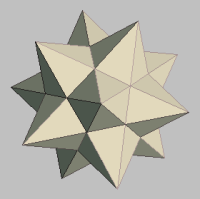MATHGOTSERVED# DMV MOTORCYCLE PRACTICE TEST

Please wait for the test to load below....

# About the Accuplacer College Algebra Mathematics Section

According to the College board "The College-Level Mathematics test measures your ability to solve problems that involve college-level mathematics concepts. There are six content areas measured on this test: (a) Algebraic Operations, (b) Solutions of Equations and Inequalities, (c) Coordinate Geometry, (d) Applications and other Algebra Topics, (e) Functions, and (f) Trigonometry. The Algebraic Operations content area includes the simplification of rational algebraic expressions, factoring and expanding polynomials, and manipulating roots and exponents. The Solutions of Equations and Inequalities content area includes the solution of linear and quadratic equations and inequalities, systems of equations, and other algebraic equations. The Coordinate Geometry content area presents questions involving plane geometry, the coordinate plane, straight lines, conics, sets of points in the plane, and graphs of algebraic functions. The Functions content area includes questions involving polynomial, algebraic, exponential, and logarithmic functions. The Trigonometry content area includes trigonometric functions. The Applications and other Algebra Topics content area contains complex numbers, series and sequences, determinants, permutations and combinations, factorials, and word problems. A total of 20 questions are administered on this test."-Source collegeboard.com